Monday, September 25, 2023

# Superelevation: Derivation, Limitations & Design

Superelevation may be defined as the transverse slope kept to neutralize the effect of centrifugal force and decrease the chances of the vehicle overturning and skidding laterally outwards by lifting the pavement’s an outside edge for the inside edge. It is usually denoted by “ e ”.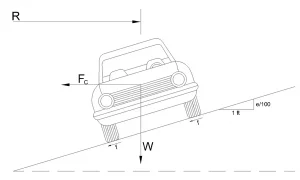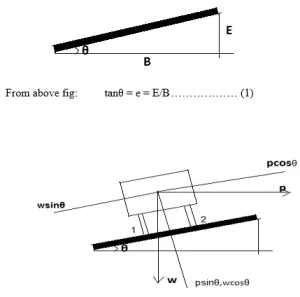Weight of Vehicle, W(↓)

Centripetal Force, P(→)

Frictional Force, F1, F2(←)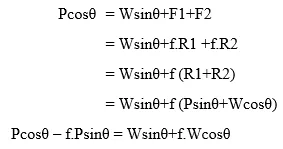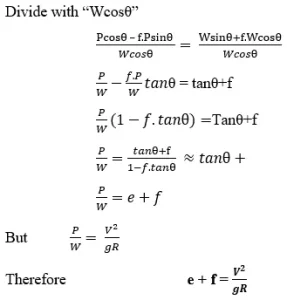Where,

e = Rate of superelevation( % )

f = Lateral friction factor ( 0.15 )

V = Velocity of vehicle in ( m/s )

g = Acceleration due to gravity = ( 9.81 m/s2 or 10 m/s)

R = Radius of circular curve ( meters )

If velocity is given in KMPH then e + f= V2/127R

### 1. Limits For Maximum and Minimum Superelevation

According to IRC, the Maximum Superelevation in Areas that are bound by Snowfall is 7% and which are not bounded by Snowfall is 10%.

Minimum superelevation = camber or cross slope Camber: Slope kept in the transverse direction to drain off rain water fastly is known as Camber or Cross slope. This will also control slipping and skidding of vehicles.

### 2. IRC Suggestions for Camber

 Type of pavement Light rainfall intensity Heavy rainfall intensity C.C pavements and thick bituminous pavements 1 in 60 1 in 50 Thin Bituminous Pavements 1 in 50 1 in 40 W.B.M and Gravel Pavements 1 in 40 1 in 33 Earthen Pavements 1 in 33 1 in25

### 3. Design of Superelevation

#### a. Step 1

Calculate the superelevation necessary for 75% design speed and assume No lateral friction is developed.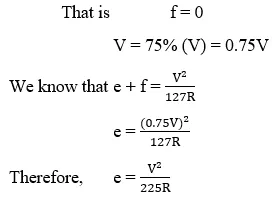If the e value is less than emax = 0.07, provide the calculated e value. Otherwise, proceed to the next step

#### b. Step 2

When ecal > emax Provide e = emax = 0.07 in this step and go to next step.

#### c. Step 3

From the above step, we have the value of e. so, a check for lateral friction factor is applied in this step for the known value of e.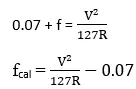If fcal < fmax (0.15) Then e = 0.07 is safe. But if fcal > 0.15 Then restrict the values to f = 0.15, e = 0.07 And go to last step.

#### c. Step 4

In this step, we will find out the value of restricted speed. Let V = Va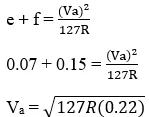If Va > V, then e = 0.07, f= 0.15. If Va < V , then also e = 0.07, f = 0.15 but, speed limitation board in the road is given which consists the value of Va.

The advantages of superelevation are as follows:

1. It makes the driver comfortable driving the vehicle.

2. It decreases the chances of the vehicle overturning.

3. It provides restriction limits for vehicles.

4. It can save from accidents that can protect a lot of lives.He is a founder and lead author of Dream Civil International and his civil engineering research articles has been taken as source by world's top news and educational sites like USA Today, Time, The richest, Wikipedia, etc.
Latest Articles

Related Articles